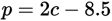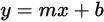# SAT Math Multiple Choice Question 782: Answer and Explanation

### Test Information

Question: 782

2. A lemonade stand's profit is given by the equation. Which of the following does the number 2 most likely represent?

• A. The price of one cup of lemonade
• B. The profit generated from the sale of one cup of lemonade
• C. The minimum number of cups of lemonade that must be sold to earn a profit
• D. The costs that must be recuperated before the lemonade stand earns any profits

Getting to the Answer: The profit equation is linear and is given in slope-intercept form (). The number 2 is the slope (m), which represents a unit rate. This means you can eliminate C and D because neither describes a rate of change. To choose between (A) and B, compare the descriptions to the equation-the 2 in the equation is the coefficient of c (which represents cups), so 2 is the price per cup of lemonade. Choice (A) is correct.